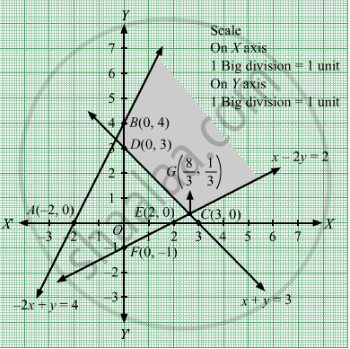# Find the Minimum Value of 3x + 5y Subject to the Constraints − 2x + Y ≤ 4, X + Y ≥ 3, X − 2y ≤ 2, X, Y ≥ 0. - Mathematics

Sum

Find the minimum value of 3x + 5y subject to the constraints
− 2x + y ≤ 4, x + y ≥ 3, x − 2y ≤ 2, xy ≥ 0.

#### Solution

First, we will convert the given inequations into equations, we obtain the following equations:
2x + y = 4, x + y = 3, x  2y = 2, x = 0 and y = 0.
The line 2x + y = 4 meets the coordinate axis at $A\left( - 2, 0 \right)$ and B(0, 4). Join these points to obtain the line 2x + y = 4.
Clearly, (0, 0) satisfies the inequation 2x + y ≤ 4. So, the region in xy-plane that contains the origin represents the solution set of the given equation.

The line x + y = 3 meets the coordinate axis at C(3, 0) and D(0, 3). Join these points to obtain the line x + y = 3.
Clearly, (0, 0) does not satisfies the inequation x + ≥ 3. So, the region in xy-plane that does not contains the origin represents the solution set of the given equation.

The line x  2y = 2 meets the coordinate axis at E(2, 0) and F(0, 1). Join these points to obtain the line x  2y = 2.
Clearly, (0, 0) satisfies the inequation x  2y ≤ 2. So, the region in xy-plane that contains the origin represents the solution set of the given equation.

Region represented by x ≥ 0 and y ≥ 0:
Since, every point in the first quadrant satisfies these inequations. So, the first quadrant is the region represented by the inequations.
These lines are drawn using a suitable scale.The corner points of the feasible region are $B\left( 0, 4 \right)$ $D\left( 0, 3 \right)$ and  $G\left( \frac{8}{3}, \frac{1}{3} \right)$ The values of Z at these corner points are as follows.

 Corner point Z = 3x + 5y $B\left( 0, 4 \right)$ 3 × 0+ 5 × 4 = 20 $D\left( 0, 3 \right)$ 3 × 0+ 5 × 3 = 15 $G\left( \frac{8}{3}, \frac{1}{3} \right)$ 3 × $\frac{8}{3}$ + 5 × $\frac{1}{3}$ = $\frac{29}{3}$

We see that the minimum value of the objective function Z is $\frac{29}{3}$  which is at $G\left( \frac{8}{3}, \frac{1}{3} \right)$ Thus, the optimal value of Z is $\frac{29}{3}$ .

Concept: Graphical Method of Solving Linear Programming Problems
Is there an error in this question or solution?

#### APPEARS IN

RD Sharma Class 12 Maths
Chapter 30 Linear programming
Exercise 30.2 | Q 24 | Page 33